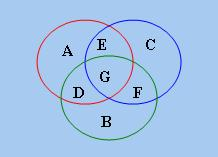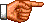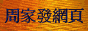# 點算的奧秘：容斥原理基本公式

「容斥原理」(Principle of Inclusion and Exclusion)(亦作「排容原理」)是「點算組合學」中的一條重要原理。但凡略為複雜、包含多種限制條件的點算問題，都要用到這條原理。現在首先從一個點算問題說起。|S1 ∪ S2 ∪ S3| = (|S1| + |S2| + |S3|) − (|S1 ∩ S2| + |S1 ∩ S3| + |S2 ∩ S3|) + |S1 ∩ S2 ∩ S3|

 |S1 ∪ S2 ∪ ... Sn| = (|S1| + |S2| + ... |Sn|) − (|S1 ∩ S2| + |S1 ∩ S3| + ... |Sn − 1 ∩ Sn|) + (|S1 ∩ S2 ∩ S3| + |S1 ∩ S2 ∩ S4| + ... |Sn − 2 ∩ Sn − 1 ∩ Sn|) − (|S1 ∩ S2 ∩ S3 ∩ S4| + ... |Sn − 3 ∩ Sn − 2 ∩ Sn − 1 ∩ Sn|) ...... + (−1)n − 1 |S1 ∩ S2 ∩ ... Sn − 1 ∩ Sn|     (1)

|S1 ∪ S2 ∪ ... Sn| = Σ1 ≤ k ≤ n(−1)k − 1 Sn, k     (2)

C(k, 1) − C(k, 2) + C(k, 3) − C(k, 4) ... (−)k − 1 C(k, k) = Σ1 ≤ r ≤ k(−1)r − 1 C(k, r)

 Σ1 ≤ r ≤ k(−1)r − 1 C(k, r) = − Σ1 ≤ r ≤ k(−1)r C(k, r) = − [Σ0 ≤ r ≤ k(−1)r C(k, r) − (−1)0 C(k, 0)] = − Σ0 ≤ r ≤ kC(k, r) (−1)r + 1

|S1 ∪ S2 ∪ ... Sn| = Σ1 ≤ k ≤ n(−1)k − 1 C(n, k) nk     (3)

|S1' ∩ S2' ∩ ... Sn'| = |U| + Σ1 ≤ k ≤ n(−1)k Sn, k     (4)

|S1' ∩ S2' ∩ ... Sn'| = |U| + Σ1 ≤ k ≤ n(−1)k C(n, k) nk     (5)

Σ1 ≤ k ≤ 5(−1)k − 1 C(5, k) (26 − k)10 = 5 × 2510 − 10 × 2410 + 10 × 2310 − 5 × 2210 + 2110

 |U| − C(3, 1) n1 + C(3, 2) n2 − C(3, 3) n3 = 6! − 3 × 5! × 2 + 3 × 4! × 22 − 1 × 3! × 23 = 720 − 720 + 288 − 48 = 240 □